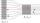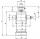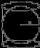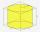Ratio + cylinder - problems

On solving problems and tasks with proportionally we recommend hint rule of three. Rule of three (proportionality) help solve examples of direct and inverse proportionality. Three members makes possible to calculate the fourth - unknown member.

1. Ratio of volumesIf the heights of two cylindrical drums are in the ratio 7:8 and their base radii are in the ratio 4:3. What is the ratio of their volumes?
2. PipesWater pipe has a cross-section 1087 cm2. An hour has passed 960 m3 of water. How much water flows through the pipe with cross-section 300 cm2 per 9 hours if water flow same speed?
3. GutterHow much metal is needed for production 46 pieces of gutter pipes with the diameter 12 cm and length of 4 m? The plate bends add 2% of the material.
4. LatheFrom the cube of edge 37 cm was lathed maximum cylinder. What percentage of the cube is left as waste after lathed?
5. Axial sectionAxial section of the cylinder has a diagonal 31 cm long and we know that the area of the side and the area of base is in ratio 3:2. Calculate the height and radius of the cylinder base.
6. Axial sectionAxial section of the cylinder has a diagonal 40 cm. The size of the shell and the base surface are in the ratio 3:2. Calculate the volume and surface area of this cylinder.
7. Velocity ratioDetermine the ratio at which the fluid velocity in different parts of the pipeline (one part has a diameter of 5 cm and the other has a diameter of 3 cm), when you know that at every point of the liquid is the product of the area of tube [S] and the fluid.
8. LatheCalculate the percentage of waste if the cube with 53 cm long edge is lathed to cylinder with a maximum volume.
9. ScaleCylinder was drawn in scale 2:1. How many times is the volume of the cylinder smaller in reality?
10. Equilateral cylinderA sphere is inserted into the rotating equilateral cylinder (touching the bases and the shell). Prove that the cylinder has both a volume and a surface half larger than an inscribed sphere.
11. Cylinder melted into cuboidA circular cylinder has area of cross section 56cm2 and the height is 10cm the cylinder is melted and made into a cuboid of base area 16cm2. What is the height of the cuboid?

We apologize, but in this category are not a lot of examples.
Do you have an interesting mathematical problem that you can't solve it? Enter it, and we can try to solve it.

To this e-mail address, we will reply solution; solved examples are also published here. Please enter e-mail correctly and check whether you don't have a full mailbox.